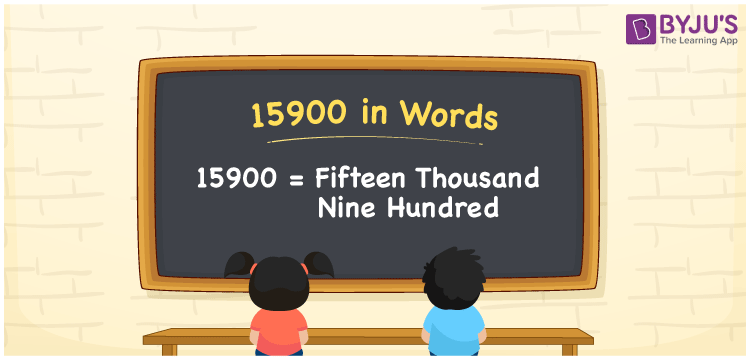# 15900 in words

15900 in words is written as Fifteen Thousand Nine Hundred . In 15900, 1 has a place value of ten thousand, 5 has a place value of thousand and 9 is in the place value of Hundred. The article on Place Value gives more information. The number 15900 is used in expressions that relate to money, distance, length, Social media view, and many more. For example, “I brought a small dictionary that has Fifteen Thousand Nine Hundred words in it.” Another example, “The EMI for Smart TV is Fifteen Thousand Nine Hundred Rupees.”

 15900 in words Fifteen Thousand Nine Hundred Fifteen Thousand Nine Hundred in Numbers 15900

## 15900 in English Words## How to Write 15900 in Words?

We can convert 15900 to words using a place value chart. The number 15900 has 5 digits, so let’s make a chart that shows the place value up to 5 digits.

 Ten thousand Thousands Hundreds Tens Ones 1 5 9 0 0

Thus, we can write the expanded form as:

1 × Ten thousand + 5 × Thousand + 9 × Hundred + 0 × Ten + 0 × One

= 1 × 10000 + 5 × 1000 + 9 × 100 + 0 × 10 + 0 × 1

= 15900

= Fifteen Thousand Nine Hundred.

15900 is the natural number that is succeeded by 15899 and preceded by 15901.

15900 in words – Fifteen Thousand Nine Hundred.

Is 15900 an odd number? – No.

Is 15900 an even number? – Yes.

Is 15900 a perfect square number? – No.

Is 15900 a perfect cube number? – No.

Is 15900 a prime number? – No.

Is 15900 a composite number? – Yes.

## Solved Example

1. Write the number 15900 in expanded form

Solution: 1 x 10000 + 5 x 1000 + 9 x 100 + 0 x 10 + 0 x 1

Or Just 1 x 10000 + 5 x 1000 + 9 x 100

We can write 15900 = 10000 + 5000 + 900 + 0 + 0

= 1 x 10000 + 5 x 1000 + 9 x 100 + 0 x1 0 + 0 x 1

## Frequently Asked Questions on 15900 in words

Q1

### How to write 15900 in words?

15900 in words is written as Fifteen Thousand Nine Hundred.
Q2

### State whether True or False. 15900 is divisible by 3?

True. 15900 is divisible by 3.
Q3

### Is 15900 divisible by 10?

Yes. 15900 is divisible by 10.